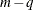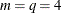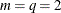### Example 58.9 Goodness-of-Fit Tests and Subpopulations

A study is done to investigate the effects of two binary factors, `A` and `B`, on a binary response, `Y`. Subjects are randomly selected from subpopulations defined by the four possible combinations of levels of `A` and `B`. The number of subjects responding with each level of `Y` is recorded, and the following DATA step creates the data set `One`:

```data One;
do A=0,1;
do B=0,1;
do Y=1,2;
input F @@;
output;
end;
end;
end;
datalines;
23 63 31 70 67 100 70 104
;
```

The following statements fit a full model to examine the main effects of `A` and `B` as well as the interaction effect of `A` and `B`:

```proc logistic data=One;
freq F;
model Y=A B A*B;
run;
```

Results of the model fit are shown in Output 58.9.1. Notice that neither the `A`*`B` interaction nor the `B` main effect is significant.

Output 58.9.1: Full Model Fit

The LOGISTIC Procedure

Model Information
Data Set WORK.ONE
Response Variable Y
Number of Response Levels 2
Frequency Variable F
Model binary logit
Optimization Technique Fisher's scoring

 Number of Observations Read 8 8 528 528

Response Profile
Ordered
Value
Y Total
Frequency
1 1 191
2 2 337

 Probability modeled is Y=1.

Model Convergence Status
Convergence criterion (GCONV=1E-8) satisfied.

Model Fit Statistics
Criterion Intercept Only Intercept and
Covariates
AIC 693.061 691.914
SC 697.330 708.990
-2 Log L 691.061 683.914

Testing Global Null Hypothesis: BETA=0
Test Chi-Square DF Pr > ChiSq
Likelihood Ratio 7.1478 3 0.0673
Score 6.9921 3 0.0721
Wald 6.9118 3 0.0748

Analysis of Maximum Likelihood Estimates
Parameter DF Estimate Standard
Error
Wald
Chi-Square
Pr > ChiSq
Intercept 1 -1.0074 0.2436 17.1015 <.0001
A 1 0.6069 0.2903 4.3714 0.0365
B 1 0.1929 0.3254 0.3515 0.5533
A*B 1 -0.1883 0.3933 0.2293 0.6321

Pearson and deviance goodness-of-fit tests cannot be obtained for this model since a full model containing four parameters is fit, leaving no residual degrees of freedom. For a binary response model, the goodness-of-fit tests havedegrees of freedom, where m is the number of subpopulations and q is the number of model parameters. In the preceding model,, resulting in zero degrees of freedom for the tests.

The following statements fit a reduced model containing only the `A` effect, so two degrees of freedom become available for testing goodness of fit. Specifying the SCALE=NONE option requests the Pearson and deviance statistics. With single-trial syntax, the AGGREGATE= option is needed to define the subpopulations in the study. Specifying AGGREGATE=(A B) creates subpopulations of the four combinations of levels of `A` and `B`. Although the `B` effect is being dropped from the model, it is still needed to define the original subpopulations in the study. If AGGREGATE=(A) were specified, only two subpopulations would be created from the levels of `A`, resulting inand zero degrees of freedom for the tests.

```proc logistic data=One;
freq F;
model Y=A / scale=none aggregate=(A B);
run;
```

The goodness-of-fit tests in Output 58.9.2 show that dropping the `B` main effect and the `A`*`B` interaction simultaneously does not result in significant lack of fit of the model. The tests’ large p-values indicate insufficient evidence for rejecting the null hypothesis that the model fits.

Output 58.9.2: Reduced Model Fit

The LOGISTIC Procedure

Deviance and Pearson Goodness-of-Fit Statistics
Criterion Value DF Value/DF Pr > ChiSq
Deviance 0.3541 2 0.1770 0.8377
Pearson 0.3531 2 0.1765 0.8382

 Number of unique profiles: 4